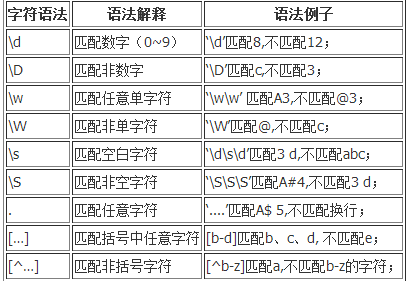﻿ 常用正则表达式 - Inzaghi-iOS开发博客,互联网资讯,米兰新闻

常用正则表达式^[1-9]\d*\$　 　 //匹配正整数
^-[1-9]\d*\$ 　 //匹配负整数
^-?[1-9]\d*\$　　 //匹配整数
^[1-9]\d*|0\$　 //匹配非负整数（正整数 + 0）
^-[1-9]\d*|0\$　　 //匹配非正整数（负整数 + 0）
^[1-9]\d*\.\d*|0\.\d*[1-9]\d*\$　　 //匹配正浮点数
^-([1-9]\d*\.\d*|0\.\d*[1-9]\d*)\$　 //匹配负浮点数
^-?([1-9]\d*\.\d*|0\.\d*[1-9]\d*|0?\.0+|0)\$　 //匹配浮点数
^[1-9]\d*\.\d*|0\.\d*[1-9]\d*|0?\.0+|0\$　　 //匹配非负浮点数（正浮点数 + 0）
^(-([1-9]\d*\.\d*|0\.\d*[1-9]\d*))|0?\.0+|0\$　　//匹配非正浮点数（负浮点数 + 0）

^[A-Za-z]+\$　　//匹配由26个英文字母组成的字符串
^[A-Z]+\$　　//匹配由26个英文字母的大写组成的字符串
^[a-z]+\$　　//匹配由26个英文字母的小写组成的字符串
^[A-Za-z0-9]+\$　　//匹配由数字和26个英文字母组成的字符串
^\w+\$　　//匹配由数字、26个英文字母或者下划线组成的字符串

“^\d+\$”　　//非负整数（正整数 + 0）
“^[0-9]*[1-9][0-9]*\$”　　//正整数
“^((-\d+)|(0+))\$”　　//非正整数（负整数 + 0）
“^-[0-9]*[1-9][0-9]*\$”　　//负整数
“^-?\d+\$”　　　　//整数
“^\d+(\.\d+)?\$”　　//非负浮点数（正浮点数 + 0）
“^(([0-9]+\.[0-9]*[1-9][0-9]*)|([0-9]*[1-9][0-9]*\.[0-9]+)|([0-9]*[1-9][0-9]*))\$”　　//正浮点数
“^((-\d+(\.\d+)?)|(0+(\.0+)?))\$”　　//非正浮点数（负浮点数 + 0）
“^(-(([0-9]+\.[0-9]*[1-9][0-9]*)|([0-9]*[1-9][0-9]*\.[0-9]+)|([0-9]*[1-9][0-9]*)))\$”　　//负浮点数
“^(-?\d+)(\.\d+)?\$”　　//浮点数
“^[A-Za-z]+\$”　　//由26个英文字母组成的字符串
“^[A-Z]+\$”　　//由26个英文字母的大写组成的字符串
“^[a-z]+\$”　　//由26个英文字母的小写组成的字符串
“^[A-Za-z0-9]+\$”　　//由数字和26个英文字母组成的字符串
“^\w+\$”　　//由数字、26个英文字母或者下划线组成的字符串
“^[\w-]+(\.[\w-]+)*@[\w-]+(\.[\w-]+)+\$”　　　　//email地址
“^[a-zA-z]+://(\w+(-\w+)*)(\.(\w+(-\w+)*))*(\?\S*)?\$”　　//url
/^(d{2}|d{4})-((0([1-9]{1}))|(1[1|2]))-(([0-2]([1-9]{1}))|(3[0|1]))\$/ // 年-月-日
/^((0([1-9]{1}))|(1[1|2]))/(([0-2]([1-9]{1}))|(3[0|1]))/(d{2}|d{4})\$/ // 月/日/年
“^([w-.]+)@(([[0-9]{1,3}.[0-9]{1,3}.[0-9]{1,3}.)|(([w-]+.)+))([a-zA-Z]{2,4}|[0-9]{1,3})(]?)\$” //Emil
“(d+-)?(d{4}-?d{7}|d{3}-?d{8}|^d{7,8})(-d+)?” //电话号码
“^(d{1,2}|1dd|2[0-4]d|25[0-5]).(d{1,2}|1dd|2[0-4]d|25[0-5]).(d{1,2}|1dd|2[0-4]d|25[0-5]).(d{1,2}|1dd|2[0-4]d|25[0-5])\$” //IP地址

http(s)?://([\w-]+\.)+[\w-]+(/[\w- ./?%&=]*)?

((25[0-5])|(2[0-4]\d)|(1\d\d)|([1-9]\d)|\d)(\.((25[0-5])|(2[0-4]\d)|(1\d\d)|([1-9]\d)|\d)){3}

(http(s)?://([\w-]+\.)+[\w-]+(/[\w- ./?%&=]*)?)|(((25[0-5])|(2[0-4]\d)|(1\d\d)|([1-9]\d)|\d)(\.((25[0-5])|(2[0-4]\d)|(1\d\d)|([1-9]\d)|\d)){3})

0～9 \d 单个数字
10～99 [1-9]\d 两位数
100～199 1\d\d 百位为1的三位数
200～249 2[0-4]\d 三位数，百位是2，十位是0～9
250～255 25[0-5] 三位数，百位是2，十位是5，个位是0～5

((25[0-5])|(2[0-4]\d)|(1\d\d)|([1-9]\d)|\d)(\.((25[0-5])|(2[0-4]\d)|(1\d\d)|([1-9]\d)|\d)){3}

[a-zA-Z0-9][-a-zA-Z0-9]{0,62}(\.[a-zA-Z0-9][-a-zA-Z0-9]{0,62})+\.?

Design By Inzaghi | 京ICP备16047555号-1